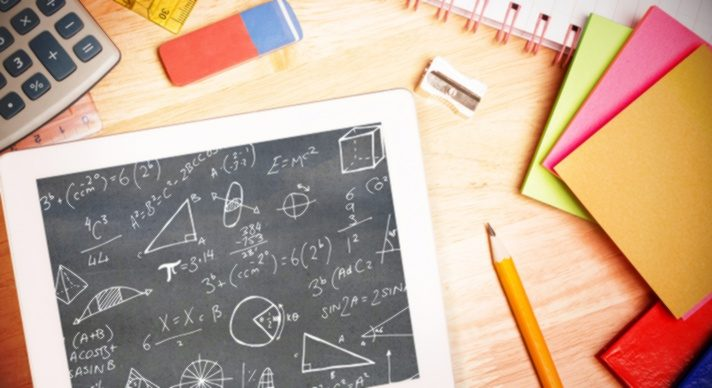# Integrated Mathematics II### Course Overview

Acellus Integrated Mathematics II, taught by Patrick Mara, is the second course of a three-part series that includes algebra, geometry, probability, and statistics. This high school math pathway is patterned after an approach typically seen internationally. Acellus Integrated Mathematics II is A-G Approved through the University of California.This course was developed by the International Academy of Science. Learn More

### Scope and Sequence

Unit 1 The introductory unit of this course provides are review of the crucial concepts of real numbers, properties, adding and subtracting, multiplying and dividing, and algebraic expressions. Unit 2 This unit discusses the definition of a function, direct variation, slope-intercept, point-slope, piece-wise defined functions, and linear models. This unit also discusses transformations of functions, absolute value functions, including how to solve them graphically, by substitution, and elimination. Unit 3 Within this unit students will learn about zero and negative exponents, multiplying powers, more multiplication properties, division properties, and rational exponents. Students will also learn about exponential functions, exponential growth and decay, and geometric sequences. Unit 4 In this unit, students will learn about adding and subtracting polynomials, multiplying and factoring, multiplying binomials, special products, and how to factor a trinomial. Students will additionally learn about factoring by grouping, and special cases that may be encountered. Unit 5 This unit guides students through quadratic graphs, quadratic functions, quadratic equations, how to solve by factoring, and completing the square. The unit will also go through the quadratic formula, complex numbers, and systems of linear and quadratic equations. Unit 6 This unit discusses polynomial functions, the fundamental theorem of algebra, math modeling, graphing square root functions, and inverse functions. Unit 7 Students will gain the knowledge of experimental versus theoretical probability in this unit.  Additionally, the concepts of probability distributions, permutations, combinations, and compound probabilities. Students will also gain the knowledge of probability models, conditional probability, and expected value. Unit 8 Students begin this unit by learning about measuring segments, the coordinate plane, inductive reasoning, conditional statements, and biconditionals, as well as deductive reasoning, and proofs. Unit 9 In this unit students will learn transversals, parallel lines, proving lines parallel, and the concepts of parallel and perpendicular.  Additionally, this unit will introduce students to the angle sum theorem. Unit 10 Within this unit students gain knowledge on congruent figures, the concepts of SSS and SAS, ASA and SAA, corresponding parts, and isosceles and equilateral triangles. Students will also gain knowledge of right triangles, and overlapping triangles. Unit 11 This unit discusses midsegments, bisectors, the center of triangles, and indirect proof.  Additionally, students will learn about inequalities in a triangle, and inequalities in two triangles. Unit 12 In this unit, students learn about polygon angle sums, parallelograms, proving a parallelogram, rhombuses, rectangles, and squares, and proving special parallelograms. Students will additionally learn about trapezoids and kites, polygons and coordinates, and using coordinates in proofs. Unit 13 This unit discusses ratios and proportions, similar polygons, and proving triangles are similar.  Also covered in this unit are the concepts of similarity in right triangles, and proportions in similar triangles. Unit 14 In this unit students will learn about pythagorean theorem, special right triangles, and trigonometry.  Additionally, the concepts of angles of elevation and depression, and pythagorean identity are introduced. Unit 15 This unit discusses areas of parallelograms and triangles, areas of trapezoids, rhombuses, and kites, as well as areas of regular polygons, and perimeters and areas of similar figures.  Additionally, this unit discusses circles and arcs, length of arcs, areas of circles and sectors, and area addition and subtraction. Unit 16 In this unit, students will gain knowledge pertaining to polyhedra, volumes of prisms and cylinders, volumes of pyramids, volumes of cones, and surface areas and volumes of spheres. Unit 17 Within this unit students will learn about tangent lines, chords and arcs, and inscribed angles, as well as angle measures and segment lengths, and circles in the coordinate plane. Unit 18 In the concluding unit of this course, students will learn about parabolas, and completing the square, as well as the concepts of distance, midpoint, and circles.